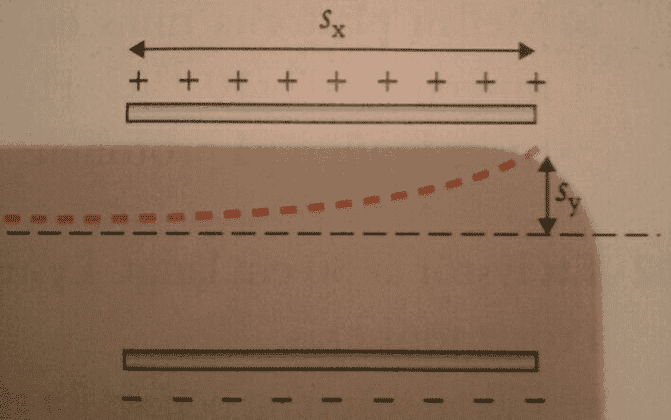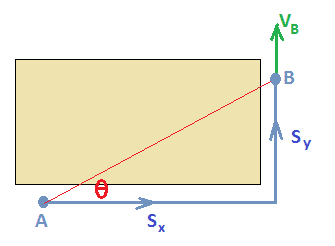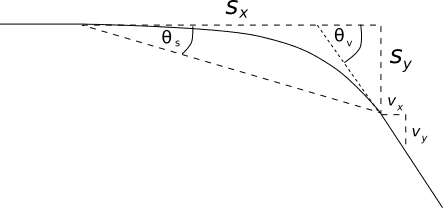# Angle of electron in an electric field

## Homework Statement

https://imgur.com/zEoCpc0I am trying to figure put the angle and my attempt is tanx=sy/sx (I have calculated a value for the distances)

But my book is saying: tanx =vy/vx

I don't understand why my way of solving it is wrong... I dont get the same answer for x :/

#### Attachments

Last edited by a moderator:

TSny
Homework Helper
Gold Member
Suppose you drive your car around a corner from point A to point B. The direction you are traveling when you get to B is given by the direction of your velocity VB at B. The angle θ, such that tanθ = sy/sx, is the angle of the red line that connects points A and B. But this is not the direction that you are traveling at B.You are calculating two different angles. Here tanθs≠tanθv. See this picture for clear explanation: